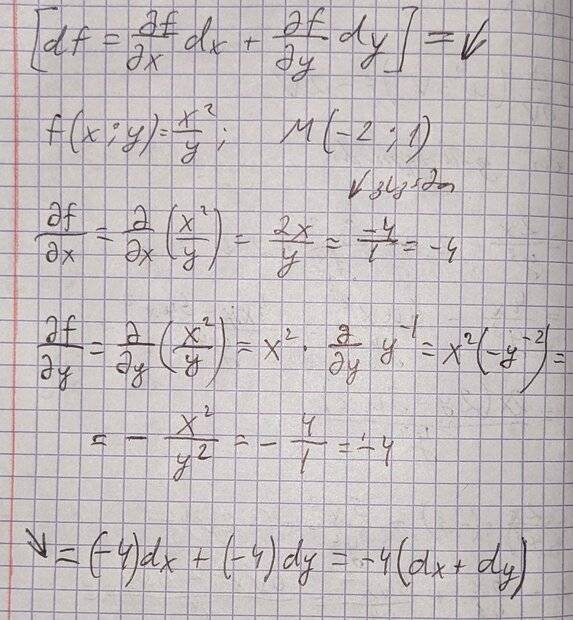# What does the differential equation answer mean?

• NODARman

#### NODARman

Homework Statement
-4(dx+dy)
Relevant Equations
What does -4(dx+dy) mean?
Hi, last semester I "solved" a full differential equation and the answer was (see the picture). What does it mean? Can I make a graphic with it or what? I really don't get it.

*Arrows are just a continuation of the main formula*I don't see a differential equation
( something of the form ##f ' = g(x,y)## )
so I have no idea what you are doing.
Can you try to explain what you think you are doing?

## \ ##

•FactChecker and topsquark
It looks like you are calculating the differential of $f(x,y) = x^2/y$ at the point $(x,y) = (-2,1)$.

•Steve4Physics and FactChecker